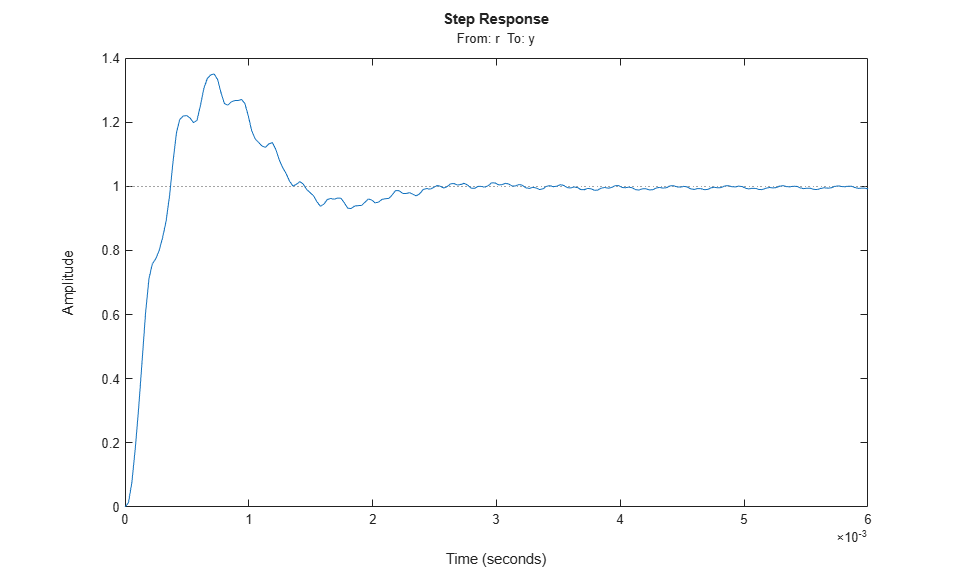## Validate the Controller Design

To validate the `hinfstruct` control design, analyze the tuned output models described in Interpret the Outputs of hinfstruct. Use these tuned models to examine the performance of the tuned system.

This example shows how to obtain the closed-loop step response of a system tuned with `hinfstruct` in MATLAB®.

You can use the tuned versions of the tunable components of your system to build closed-loop or open-loop numeric LTI models of the tuned control system. You can then analyze open-loop or closed-loop performance using other Control System Toolbox™ tools.

In this example, create and analyze a closed-loop model of the HDA system tuned in Tune the Controller Parameters. To do so, use `getIOTransfer` to extract from the tuned control system the transfer function between the step input and the measured output.

```Try = getIOTransfer(T,'r','y'); step(Try)```This example shows how to write tuned values to your Simulink® model for validation.

The `slTuner` interface linearizes your Simulink model. As a best practice, validate the tuned parameters in your nonlinear model. You can use the `slTuner` interface to do so.

In this example, write tuned parameters to the `rct_diskdrive` system tuned in Tune the Controller Parameters.

Make a copy of the `slTuner` description of the control system, to preserve the original parameter values. Then propagate the tuned parameter values to the copy.

```ST = copy(ST0); setBlockValue(ST,T); ```

This command writes the parameter values from the tuned, weighted closed-loop model `T` to the corresponding parameters in the interface `ST`.

You can examine the closed-loop responses of the linearized version of the control system represented by `ST`. For example:

```Try = getIOTransfer(ST,'r','y'); step(Try) ```Since `hinfstruct` tunes a linearized version of your system, you should also validate the tuned controller in the full nonlinear Simulink model. To do so, write the parameter values from the `slTuner` interface to the Simulink model.

```writeBlockValue(ST) ```

You can now simulate the model using the tuned parameter values to validate the controller design.